# How to Divide in Excel: Division Formulas and Shortcuts

Posted April 16, 2019 by Sheraz Ali in Microsoft ExcelThere are many different methods to divide in excel. Some of them require a lot of time and manual labor, whereas others are more to simple to follow.

In this tutorial, we will focus on the latter so that basic functions in Excel, such as additions, subtractions, multiplications, and in this case, divisions are refined for your convenience.

### Important Things to Know to Divide Numbers in Excel

Before we jump on to the actual process of division, there are some things that you need to know. Most of them involve the protocols associated with Excel Formulae.

• In Excel, formulae always start with an equal (=) sign.
• You have to type this equal sign in the cell you want the formula to operate in.
• You have to insert the slash sign (/) between two dividing numbers.
• After inserting the formula for division, you have to hit ENTER your keyboard for it to give the final result.

### Method 1: For Dividing Within a Cell

If you want to divide two random numbers in a single cell and aren’t dealing with rows of data, here’s what you have to do.

1. Open your Excel Spreadsheet.

2. Click an empty cell reference.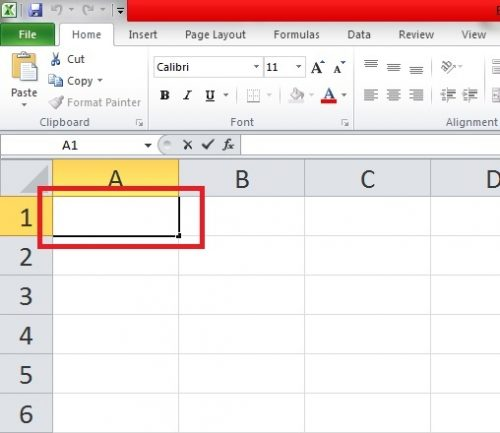3. Type an equal (=) sign.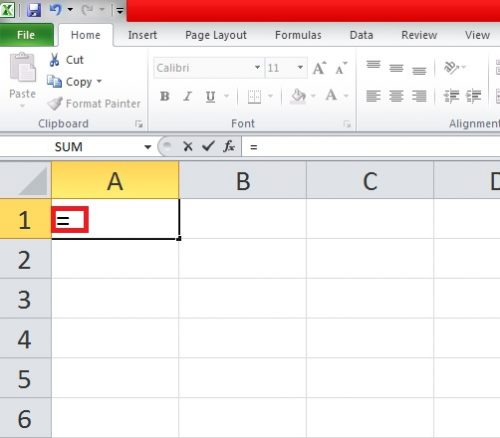4. Type the two numbers you want to divide separated with a slash (/) symbol. In the case, I’m dividing the number 1456 by 45.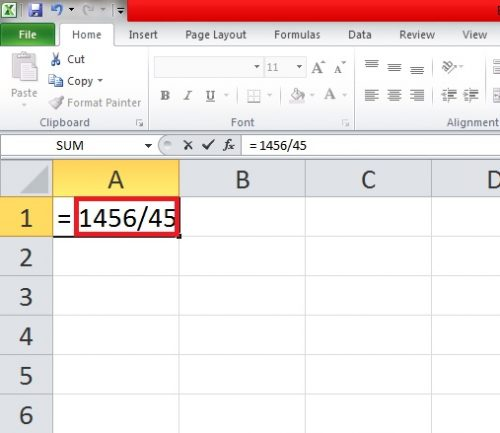5.  Hit Enter.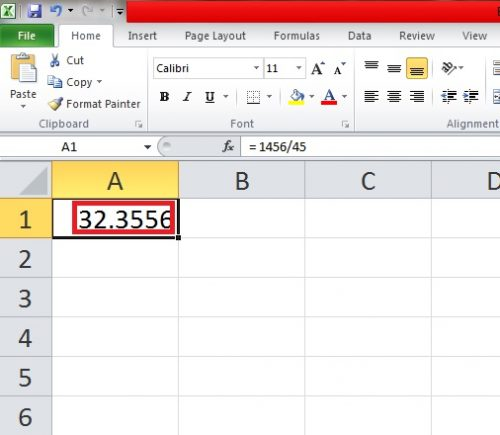### Method 2: For Dividing Through Cell References

In the case of data with a lot of numbers, you can use the names of cell references (A2, B3, C4, etc. etc.) to refer to the numbers that you want to divide to get more accurate results. Here’s how this works…

1. Open the Excel Spreadsheet with your data.

2. Click on an empty cell. Any cell will suffice.

3. Start your formula with an equal (=) sign.

4.  Write the names of the cell references that you want to divide separated by a slash symbol (/). I’m dividing the following references and my final formula will come out like this: = A2/A3/A5.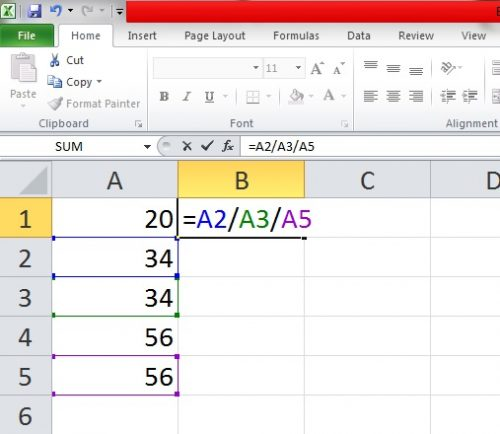Hit Enter.

### Method 3: For Dividing with a Constant Number

You can also divide a column of data with the same number in Excel. For example, if you have a vertical assortment of numbers and you want to divide them all with one number — say 100 — here’s how you can do that.

1. Click and Empty Cell.

2. Insert an equal (=) sign.

3. Type the name of the first number in the column you want to divide the constant number with. In this case, I’m dividing the number in cell reference A1 with the constant number 100, so I’ll type it after the equal sign: =A1.

4.  Followed by a slash symbol (/), write a \$ sign, which in turn will be followed by the name of the cell reference of the constant number like this with one more dollar sign in between like this: A1/\$B\$1.5.  Drag the cell reference C1 down to divide the rest of the column.

And that’s it. This is all you need to know to perform all kinds of division operations in Microsoft Excel. For further assistance, check these related techs-recipes. They will serve to polish your skills of this enormously popular spreadsheet software.

How to Remove Duplicates in Excel

How to Add Drop-Down List in Excel

### About Sheraz Ali

An established copywriter, with a longstanding experience in a vast array of industries, including but not limited to spirituality, technology, cannabis and travel.
View more articles by Sheraz Ali

#### The Conversation

Follow the reactions below and share your own thoughts.